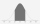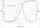Component fail

There is a 90 percent chance that a particular type of component will perform adequately under high temperature conditions. If the device involved has four such components, determine the probability that the device is inoperable because exactly one of the four components fails

Result

p =  70.84 %

Solution:Leave us a comment of example and its solution (i.e. if it is still somewhat unclear...):Be the first to comment!To solve this example are needed these knowledge from mathematics:

Looking for a statistical calculator? Our percentage calculator will help you quickly calculate various typical tasks with percentages. Would you like to compute count of combinations?

Next similar examples:

1. TV failThe TV has after 10,000 hours average 25 failures. Determine the probability of TV failure after 200 hours of operation.
2. Left handedIt is known that 25% of the population is left-handed. What is the probability that there is a maximum of three left-handers at a seminar where there are 30 participants?
3. Family94 boys are born per 100 girls. Determine the probability that there are two boys in a randomly selected family with three children.
4. Boys and girlsThere are 11 boys and 18 girls in the classroom. Three pupils will answer. What is the probability that two boys will be among them?
5. Families 2There are 729 families having 6 children each. The probability of a girl is 1/3 and the probability of a boy is 2/3. Find the the number of families having 2 girls and 4 boys.
6. SalesFrom statistics of sales goods, item A buy 51% of people and item B buys 59% of people. What is the probability that from 10 people buy 2 item A and 8 item B?
7. Internet anywhereIn school, 60% of pupils have access to the internet at home. A group of 8 students is chosen at random. Find the probability that a) exactly 5 have access to the internet. b) At least 6 students have access to the internet
8. DiceWe throw 10 times a play dice, what is the probability that the six will fall exactly 4 times?
9. DiceWe throw five times the dice. What is the probability that six fits exactly twice?
10. TestThe teacher prepared a test with ten questions. The student has the option to choose one correct answer from the four (A, B, C, D). The student did not get a written exam at all. What is the probability that: a) He answers half correctly. b) He answers.
11. Distribution functionX 2 3 4 P 0.35 0.35 0.3 The data in this table do I calculate the distribution function F(x) and then probability p(2.5